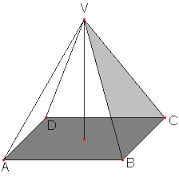The regular quadrilateral pyramid has a surface of 260 cm2 and a side wall area of 40 cm2. Calculate the length of the base edge and the wall height.

a =  10 cm
h2 =  8 cm

### Step-by-step explanation:Did you find an error or inaccuracy? Feel free to write us. Thank you!

Tips for related online calculators
Need help calculating sum, simplifying, or multiplying fractions? Try our fraction calculator.

#### Grade of the word problem:

We encourage you to watch this tutorial video on this math problem: